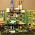# Fifo depth calculation

Assuming,
• F1 = frequency of the writing side.
• F2 = frequency of the reading side.
• D = data burst.

Burst duration = D/F1
Data Rec'd, Rx= (D/F1) * F2, assuming simultaneous read for the duration.
Extra storage during FULL condition, Backlog = D-Rx = D(F1-F2)/F1

To accommodate latency or response time in the receiver, T, we need additional T * F1 locations.
Receiver also needs time to read all this backlog, so the idle time between bursts must be long enough. So this minimum time is called mop-up time = backlog/F2 = D * (F1-F2)/(F1.F2)

Note: For bursts of data which are written for partial amount time for a given number of cycles and read that are happening continously "or" are also read for a partial amount of time for a given number of cycles, the calculation has to account for the next burst.

1.if i take f1=100 and f2=20
then if burst is 100
according to formula i get 4 ...
If i take f1=20 f2=100
then how to claculate f1-f2 is -ve...

2.Hi Anonymous:
4 is wrong, 8 is correct.

If your reading side is faster than writing, then you get an underflow condition.... In this condition, why do you need to calculate fifo depth?

3.Please can anyone explain this logic with an example...........it would be helpful to me..